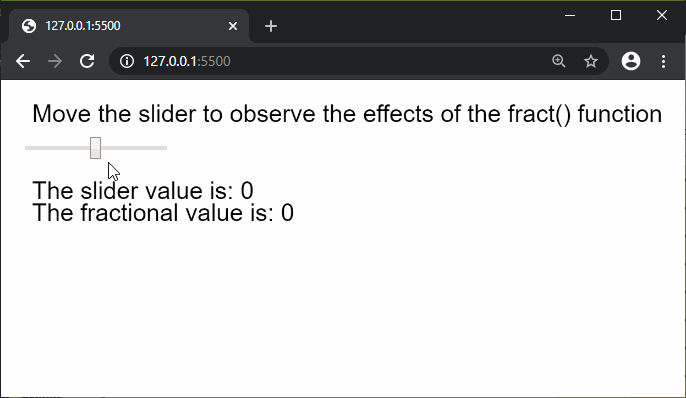# p5.js | fract() Function

The fract() function in p5.js is used to find the fractional part of a number. It can be mathematically represented as {x} where ‘x’ is the number.

Syntax:

`fract( num )`

Parameters: This function accepts single parameter as mentioned above and described below.

• num: This is a number of which the fractional part is to be found out.

Return Value: It returns a number with the fractional part of the given number.

Below example illustrates the fract() function in p5.js:

Example:

 `function` `setup() { ` `  ``createCanvas(600, 200); ` `  ``textSize(22); ` `  `  `  ``text(``"Move the slider to observe the effects"``+ ` `       ``" of the fract() function"``, 20, 30); ` `  `  `  ``sliderElem = createSlider(-5, 5, 0, 0.0001); ` `  ``sliderElem.position(20, 50); ` `} ` `  `  `function` `draw() { ` `  ``clear(); ` `  ``text(``"Move the slider to observe the"``+ ` `       ``" effects of the fract() function"``, 20, 30); ` `  `  `  ``sliderVal = sliderElem.value(); ` `  `  `  ``// Find the fractional part of the number ` `  ``fractionalVal = fract(sliderVal); ` `  `  `  ``text(``"The slider value is: "` `+ sliderVal, 20, 100); ` `  ``text(``"The fractional value is: "` `+ fractionalVal, 20, 120); ` `} `

Output:Online editor: https://editor.p5js.org/

Reference: https://p5js.org/reference/#/p5/fractMy Personal Notes arrow_drop_upCheck out this Author's contributed articles.

If you like GeeksforGeeks and would like to contribute, you can also write an article using contribute.geeksforgeeks.org or mail your article to contribute@geeksforgeeks.org. See your article appearing on the GeeksforGeeks main page and help other Geeks.

Please Improve this article if you find anything incorrect by clicking on the "Improve Article" button below.

Article Tags :

Be the First to upvote.

Please write to us at contribute@geeksforgeeks.org to report any issue with the above content.